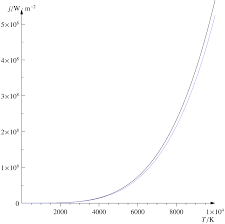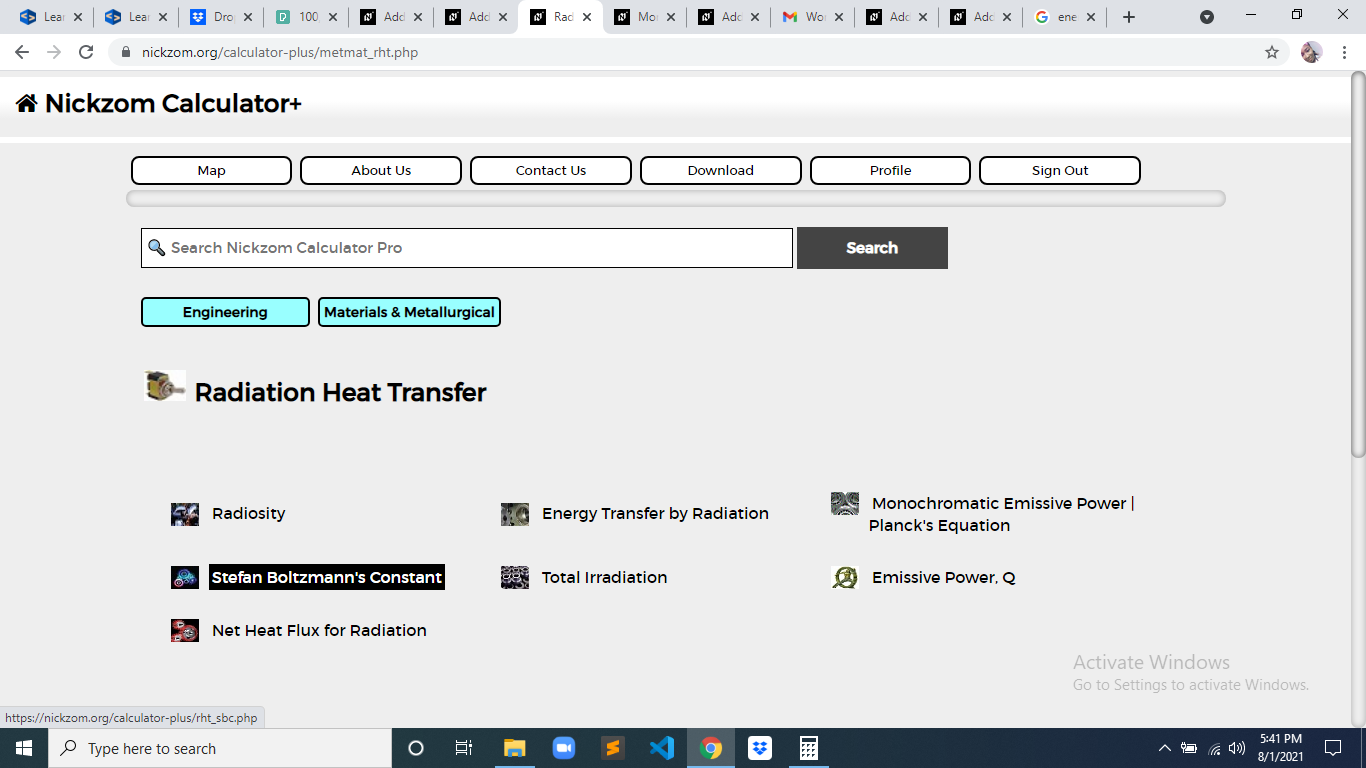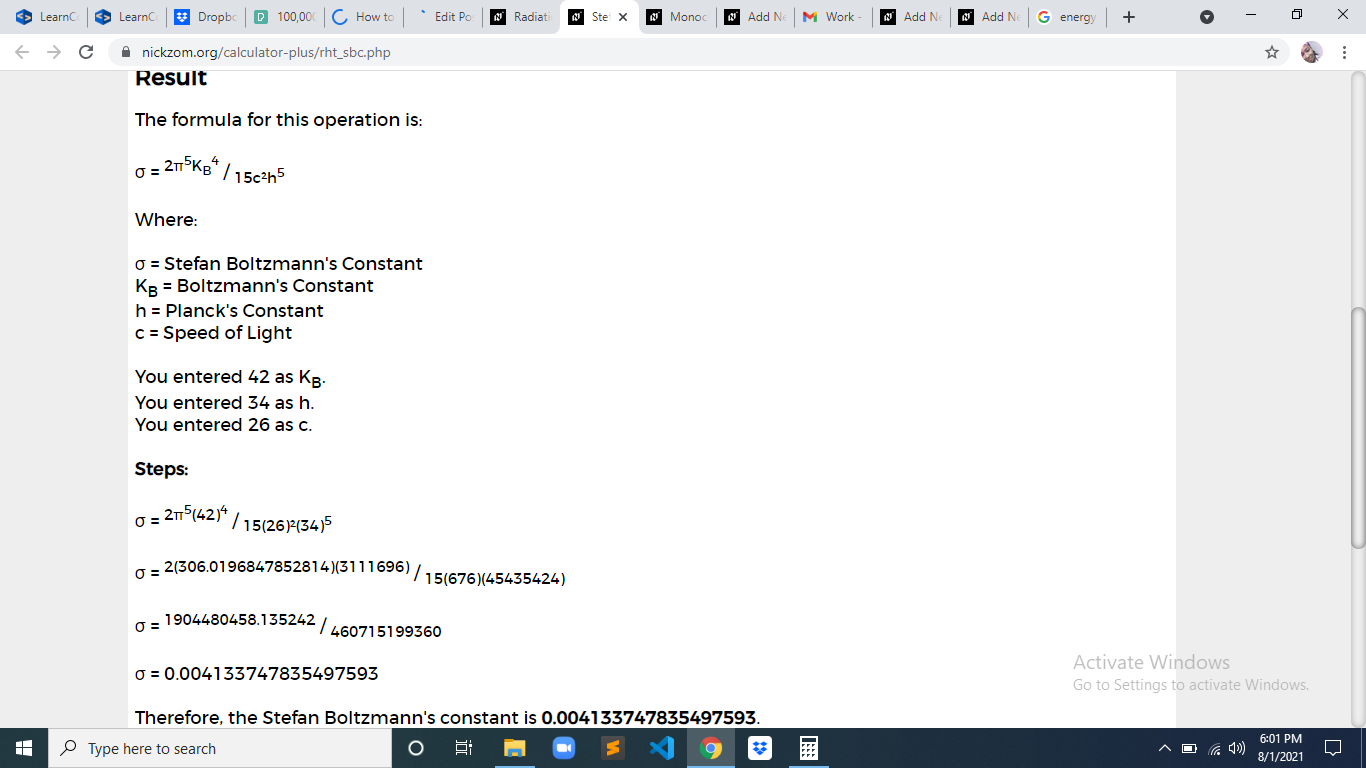# How to Calculate and Solve for Stefan Boltzmann’s Constant | Radiation Heat TransferThe image above represents stefan boltzmann’s constant.

To compute for stefan boltzmann’s constant, three essential parameters are needed and these parameters are Boltzmann’s Constant (KB), Planck’s Constant (h) and Speed of Light (c).

The formula for calculating stefan boltzmann’s constant:

σ = 2π5KB4 / 15c²h5

Where:

σ = Stefan Boltzmann’s Constant
KB = Boltzmann’s Constant
h = Planck’s Constant
c = Speed of Light

Let’s solve an example;
Find the stefan boltzmann’s constant when the boltzmann’s constant is 42, the planck’s constant is 34 and the speed of light is 26.

This implies that;

KB = Boltzmann’s Constant = 42
h = Planck’s Constant = 34
c = Speed of Light = 26

σ = 2π5KB4 / 15c²h5
σ = 2π5(42)4 / 15(26)²(34)5
σ = 2(306.019)(3111696) / 15(676)(45435424)
σ = 1904480458.135 / 460715199360
σ = 0.00413

Therefore, the stefan boltzmann’s constant is 0.00413.

Nickzom Calculator – The Calculator Encyclopedia is capable of calculating the stefan boltzmann’s constant.

To get the answer and workings of the stefan boltzmann’s constant using the Nickzom Calculator – The Calculator Encyclopedia. First, you need to obtain the app.

You can get this app via any of these means:

To get access to the professional version via web, you need to register and subscribe for NGN 2,000 per annum to have utter access to all functionalities.
You can also try the demo version via https://www.nickzom.org/calculator

Apple (Paid) – https://itunes.apple.com/us/app/nickzom-calculator/id1331162702?mt=8
Once, you have obtained the calculator encyclopedia app, proceed to the Calculator Map, then click on Materials and Metallurgical under Engineering.Now, Click on Radiation Heat Transfer under Materials and MetallurgicalNow, Click on Stefan Boltzmann’s Constant under Radiation Heat TransferThe screenshot below displays the page or activity to enter your values, to get the answer for the stefan boltzmann’s constant according to the respective parameter which is the Boltzmann’s Constant (KB), Planck’s Constant (h) and Speed of Light (c).Now, enter the values appropriately and accordingly for the parameters as required by the Boltzmann’s Constant (KB) is 42, Planck’s Constant (h) is 34 and Speed of Light (c) is 26.Finally, Click on CalculateAs you can see from the screenshot above, Nickzom Calculator– The Calculator Encyclopedia solves for the stefan boltzmann’s constant and presents the formula, workings and steps too.